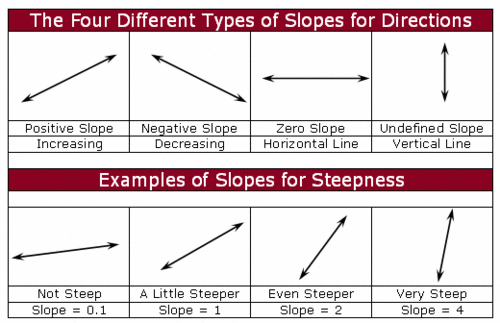RRMS | Writing Equations from Two Points
3 years ago
elliottmbetrand
Save
Edit
Host a game
Live GameLive
Homework
Solo Practice
Practice11 QuestionsShow answers
• Question 1
900 seconds
Q. Find the equation of the line that passes through the points:

(-2, 4) and (1, 10)
y = 2x - 1
y = -2x + 1
y = 2x
y = -2x
• Question 2
900 seconds
Q. Find the equation of the line that passes through the points:

(2, 2) and (3, 4)
y = 2x + 2
y = -2x - 2
y = -2x + 2
y = 2x - 2
• Question 3
900 seconds
Q. Find the equation of the line that passes through the points:

(-3, 3) and (3, -1)
y = -⅔ x + 1
y = -⅔ x
y = ⅔ x + 1
y = -⅔ x +3
• Question 4
900 seconds
Q. Find the equation of the line that passes through the points:

(3, 4) and (0, -5)
y = 3x + 5
y = -3x + 4
y = -3x + 4
y = 3x - 5
• Question 5
900 seconds
Q. Find the equation of the line that passes through the points:
(0, -2) and (-5, 3)
y = -x - 2
y = x - 2
y = x + 3
y = -x - 3
• Question 6
900 seconds
Q. Find the equation of the line that passes through the points:
(-4, -2) and (-3, 5)
y = 1/7 x + 26
y = 7x + 26
y = -7x + 26
y = -7x - 26
• Question 7
900 seconds
Q. Find the equation of the line that passes through the points:
(4, -1) and (0, -3)
y = 2x - 3
y = -2x + 3
y = ½ x - 3
y = -½ x + 3
• Question 8
900 seconds
Q. Find the equation of the line that passes through the points:
(3, 3) and (1, -5)
y = -9x + 4
y = 4x - 9
y = ¼ x + 9
y = -9x - 4
• Question 9
900 seconds
Q. Find the equation of the line that passes through the points:
(5, 3) and (4, -3)
y = 27x + 6
y = -6x
y = 6x - 27
y = 6x + 27
• Question 10
900 seconds
Q. Find the equation of the line that passes through the points:
(0, -5) and (4, 3)
y = 2x - 5
y = 2x + 5
y = 5x - 2
y = 5x + 2
• Question 11
900 seconds
Q. Identify the slope and y-intercept of the equation below:
y = -3x + 5